ISEE Lower Level Math : Ratio and Proportion

Example Questions

Example Question #6 : How To Find A Proportion

A bag of marbles contains 4 green marbles, 7 black marbles, and 5 red marbles. What is the proportion of green marbles?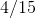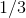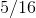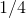Explanation:

First, sum the total number of marbles: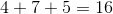Next, divide the total number of green marbles by the total number of marbles and simplify: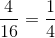Example Question #522 : Isee Lower Level (Grades 5 6) Mathematics Achievement

A bag has 11 green chips, 7 blue chips, and 6 red chips. What is the proportion of green chips?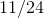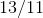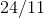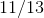Explanation:

The proportion is determined by dividing the number of selected items by the total number of items.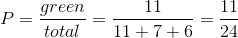Example Question #8 : How To Find A Proportion

A drawer contains 5 pairs of blue socks, 2 pairs of white socks, 4 pairs of brown socks, and 7 pairs of black socks. What is the proportion of brown socks?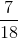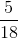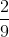Explanation:

A proportion is determined by dividing the number of a specific event by the total number of events: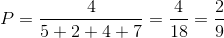Example Question #9 : How To Find A Proportion

If Eric can read a page from his text book inminutes, how many pages can he read in 15 minutes?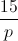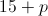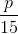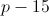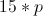Explanation:

The problem can be represented by the proportion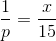.

Solve: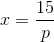Eric can read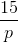pages in 15 minutes.

Example Question #10 : How To Find A Proportion

If Kristin makes $300 in a week when she works 15 hours, how much would she make if she worked 24 hours next week at the same rate? Possible Answers: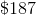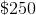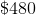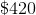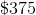Correct answer:Explanation: To find the rate at which she is currently paid, divide$300 by the hours she works, 15, to see that she earns $20 per hour. To find her earnings for next week, multiply 24 hours by$20 per hour to see that she will make \$480 next week.

Or set up the proportion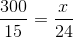and solve for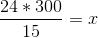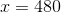Example Question #531 : Numbers And Operations

There are 24 students in Mrs. Brown's classroom. 6 of them have red backpacks. What percentage of the students have red backpacks?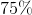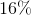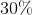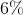Explanation:

Let's first determine the fraction of students in the class that have red backpacks. We can then convert the fraction to a percentage.

The question tells us that 6 out of 24 students have red backpacks. This is equal to six over twenty-four: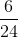We can reduce the fraction by removing a common factor.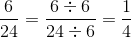The percentage that is equivalent tois 25 percent, or.

Therefore,is the correct answer.

Example Question #41 : Ratio And Proportion

Megan buys cookie packs that come in either small or large sizes. The small size has 3 vanilla cookies and 2 chocolate cookies. The large size has the same proportion of vanilla and chocolate cookies. The large comes with 6 chocolate cookies. How many vanilla cookies are in the large box?Explanation:

We know that there are the same proportions of cookies in both the small and large boxes.

Let's start by looking at the small box. We know that there are 3 vanilla and 2 chocolate cookies, or a ratio of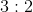.

In the large box, we know there are 6 chocolate cookies. Ifis the number of vanilla cookies, then the ratio in the large box is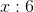.

The ratios must be equal.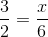Cross-multiply and solve for.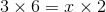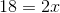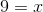Example Question #531 : Numbers And Operations

The ratio of men to women in a room is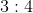. If the room has 21 people, and 2 men then leave the room, what is the proportion of men in the room?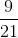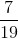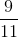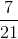Explanation:

If there is a room of 21 people in which the ratio of men to women is, that means that there are 9 men and 12 women in the room.

If 2 men leave the room, there will be 7 men and 12 women in the room, with a total of 19 people.

Therefore, the proportion of the men in the room isExample Question #534 : Numbers And Operations

If the ratio of boys to girls in a class is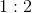, what fraction of the class is boys?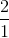Explanation:

If the ratio of boys to girls in a class is, that means that for every 3 students, 1 is a boy.

Looking at the ratio, we see that there is one boy for every two girls. In a group of three students, you would have one boy and two girls.

We can write the fraction of boys as the number of boys over the number of students in the group.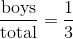Example Question #535 : Numbers And Operations

If it takes Linda 6 minutes to complete a multiple choice question, then what percentage of a 10 question multiple choice test will she complete in half an hour?If it takes Linda 6 minutes to complete a multiple choice question, then she will complete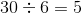questions in 30 minutes.